Glencoe Geometry: Online Textbook Help Final Exam

Free Practice Test Instructions:

Choose your answer to the question and click 'Continue' to see how you did. Then click 'Next Question' to answer the next question. When you have completed the free practice test, click 'View Results' to see your results. Good luck!

Answered 0 of 15

Question 2 2. What is the area of the shaded area, rounded to the nearest whole number?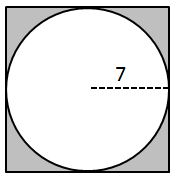Question 4 4. Which of the following represents the angle bisector theorem for the pictured triangle?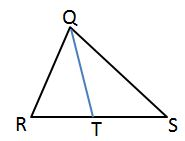Question 9 9. In the pictured triangle, side a is 32, side b is 24, and side c is 37. What is the approximate measure of ∠C? When calculating the inverse cosine, round the initial value to the nearest whole number.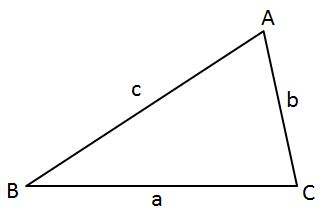Question 10 10. What is the volume of this prism?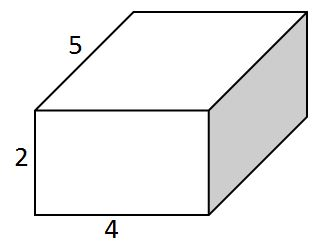Question 11 11. What is the area of the pictured rectangle?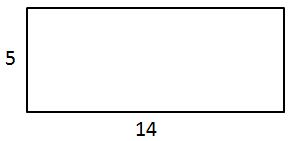Question 12 12. How can we explain that the pictured triangles are congruent?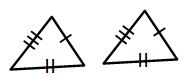Question 13 13. What type of line segment is represented by the dotted line in the pictured triangle?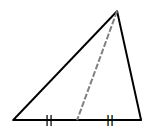Question 15 15. Find the perimeter.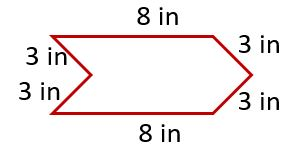Are you a student or a teacher?

Glencoe Geometry: Online Textbook Help Final Free Practice Test Instructions

Choose your answer to the question and click 'Continue' to see how you did. Then click 'Next Question' to answer the next question. When you have completed the free practice test, click 'View Results' to see your results. Good luck!

Support